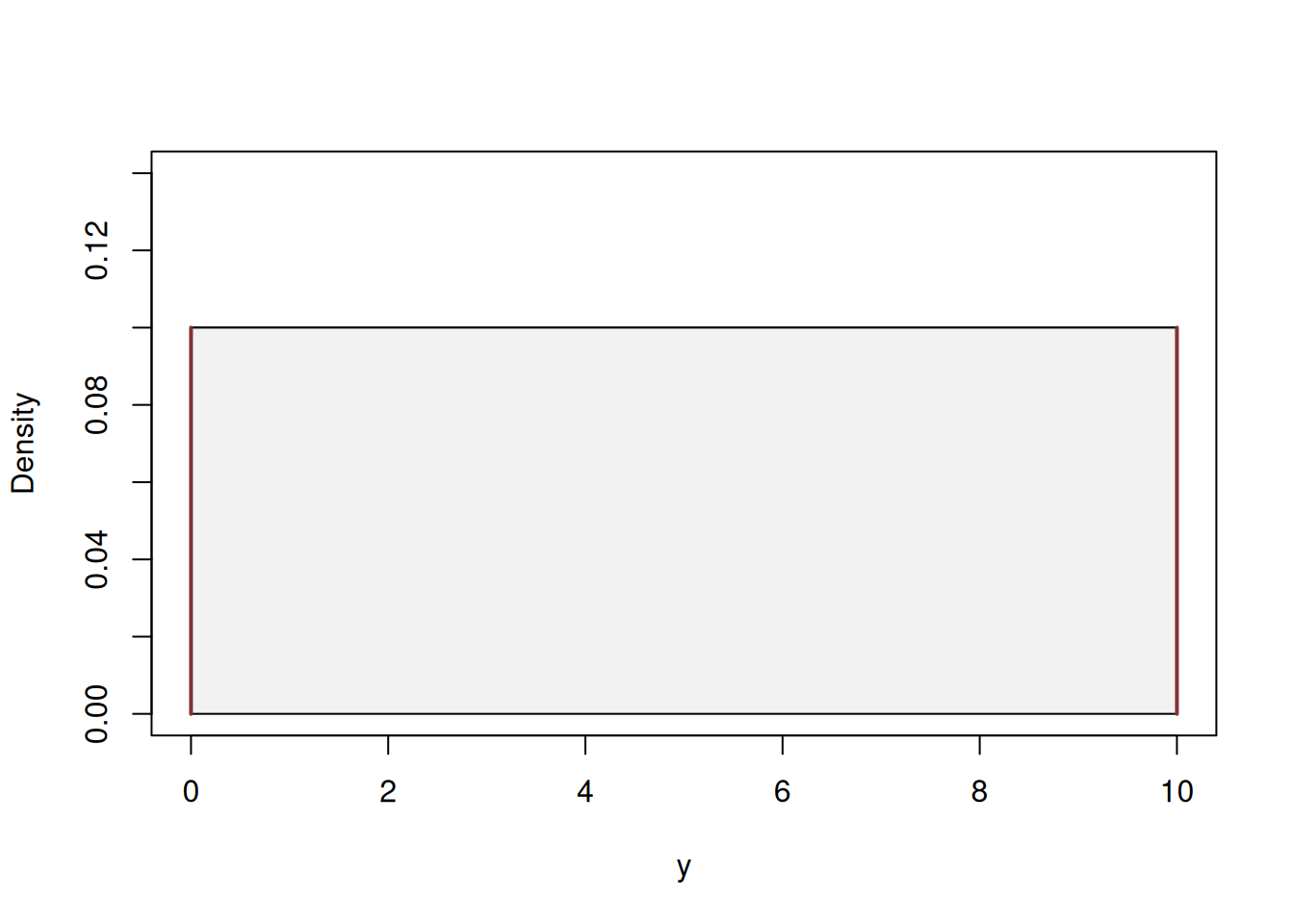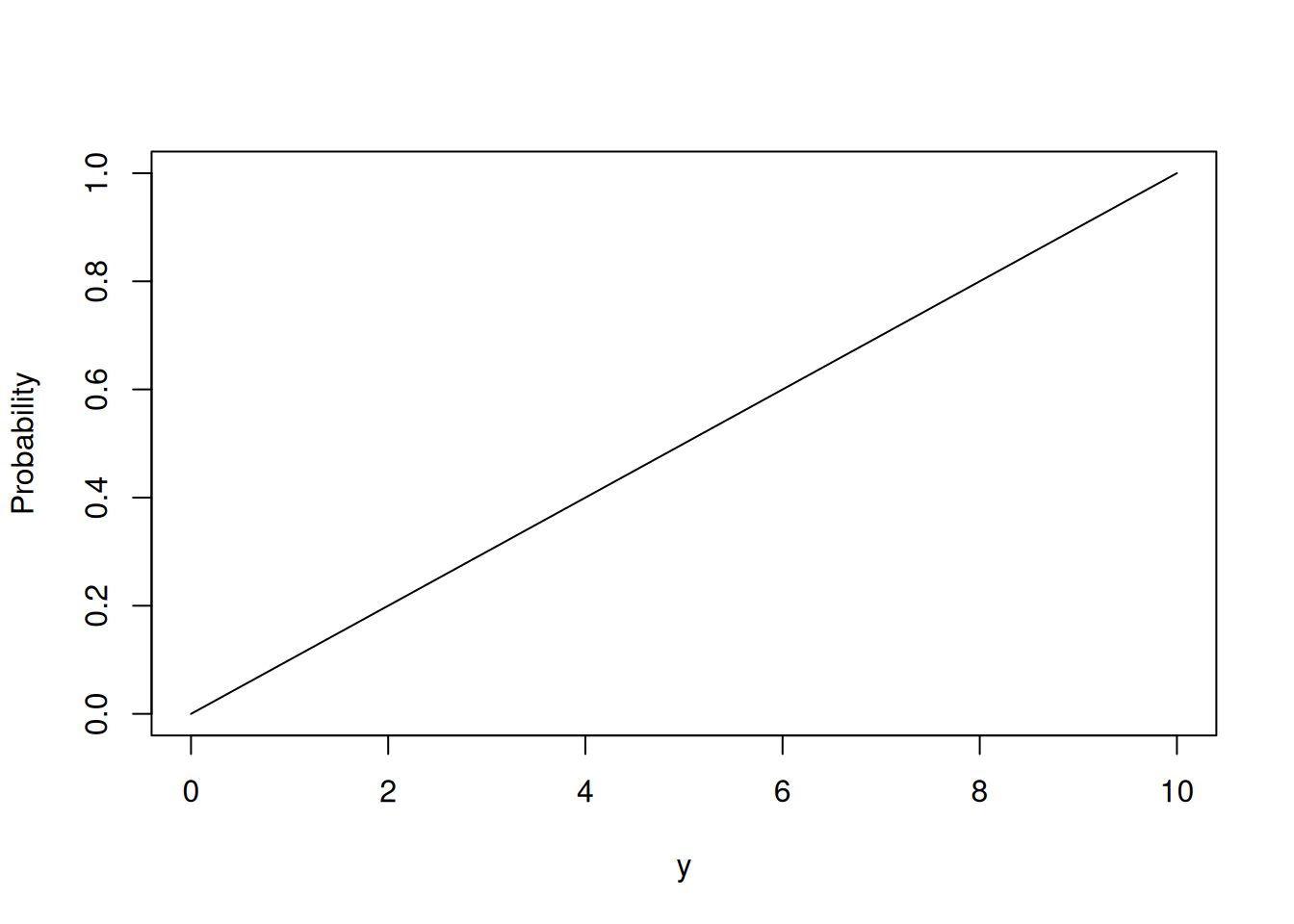This book is in Open Review. I want your feedback to make the book better for you and other readers. To add your annotation, select some text and then click the on the pop-up menu. To see the annotations of others, click the button in the upper right hand corner of the page

## 4.2 Continuous Uniform distribution

One of the simplest continuous distributions used in statistics is the uniform one. It has similarities with the discrete one, which was discussed in Section 3.5, but due to the different nature of the random variable is parameterised differently. First, because we are discussing continuous variable, it is always defined on a segment of values, from $$a$$ to $$b$$. For example, we can have a random variable which can take any value from 0 to 10 with equal likelihood. Mathematically, the PDF of continuous distribution can be written as: \begin{equation} f(y, b, a) = \left\{\begin{aligned} & \frac{1}{b-a} & \text{ for } y \in [a, b] \\ & 0 & \text{ otherwise } \end{aligned} \right. . \tag{4.1} \end{equation} It can be represented visually as shown in Figure 4.6.Figure 4.6: Probability Density Function of Continuous Uniform distribution.

According to this distribution, it is equally likely to have 1, 1.1, 1.0001, 9 etc values. The mean of this distribution coincides with the middle of the segment and can be calculated as: $\begin{equation} \mathrm{E}(y) = \frac{1}{2}(a+b) , \tag{4.2} \end{equation}$ while the variance is calculated as: $\begin{equation} \mathrm{V}(y) = \frac{1}{12}(b-a)^2 . \tag{4.3} \end{equation}$ The CDF of the Uniform distribution corresponds to the straight line going from the point (a,0) to the point (b,1), as shown in Figure 4.7.Figure 4.7: Cumulative Density Function of Continuous Uniform distribution.

Mathematically, the CDF can be represented as: \begin{equation} F(y, b, a) = \left\{\begin{aligned} & 0 & \text{ if } y<a \\ & \frac{y-a}{b-a} & \text{ for } y \in [a, b] \\ & 1 & \text{ if } y>b \end{aligned} \right. . \tag{4.4} \end{equation}

Continuous uniform distribution is sometimes used in statistics as a prior, when a researcher does not have grounds to assume any other, more complicated distribution.

In R, this distribution is implemented in stats package with functions dunif(), punif(), qunif() and runif() for PDF, CDF, QF and random generator respectively.